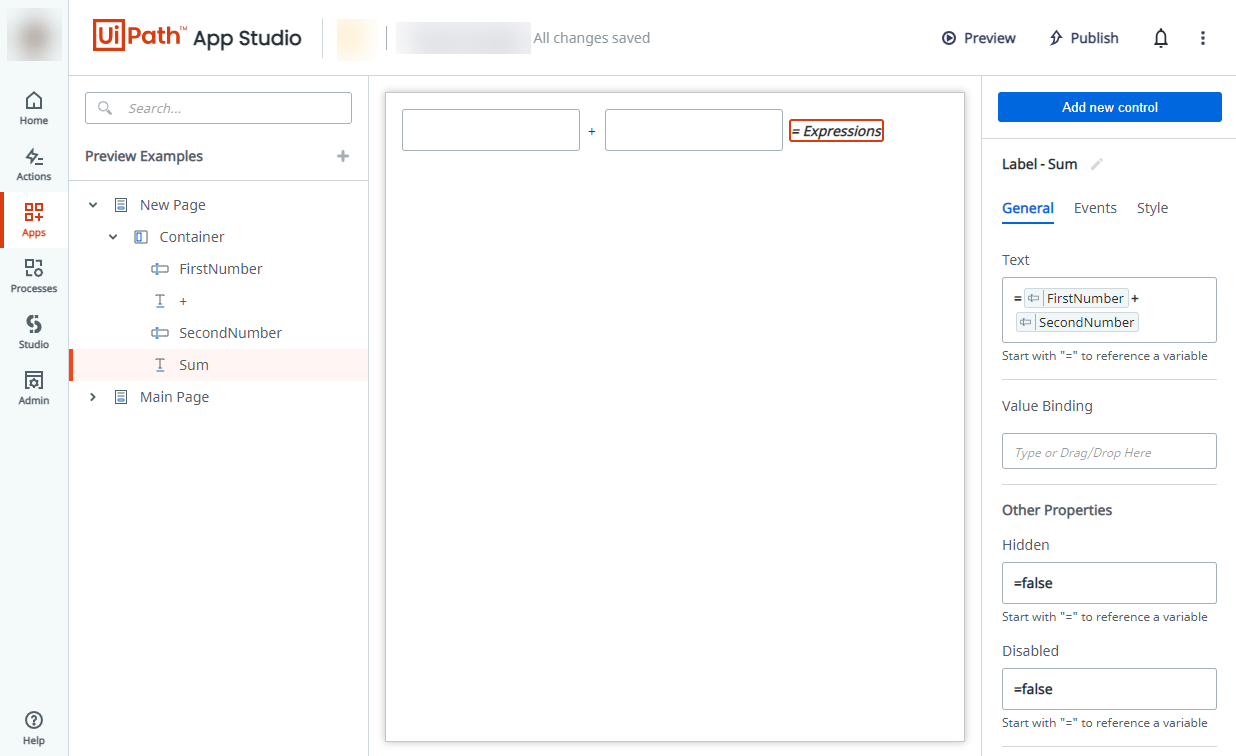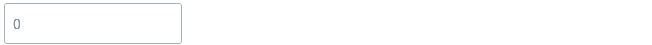Subscribe

# Using Expressions

To better understand how expressions and operators can be chained together to create more complex behaviors, follow the examples below.

# Calculator

To build a simple calculator, follow the procedure below.

StepAction
2Add two textboxes with a label in between.
Change the name of the controls as follows:
First textbox: `FirstNumber`
Label: `+`
Second texbox: `SecondNumber`
3Add another label after the second textbox.
Change the name to `Sum`.
4Add the following expression to the `Sum` label:
= `FirstNumber` + `SecondNumber`

After following the procedure above, the app will look as in the image below.# Input validation

To build a simple input validation, follow the procedure below.

StepAction
2Create an app variable called `Number`.
3Create a textbox that is value bound to the `Number` variable.
Add the default text `0`.
4Add a label with the following text: `This value is greater than or equal to 5!`.
=`Number` <5

When the inserted number is lower than five, the validation label is hidden. After following the procedure above, the runtime should look like below:# Sum of a column

To return the sum of a specified column of a table bound to Orchestrator output, follow the procedure below.
In this example we will retrieve the sum of a column called Age, containing the age of all people listed in the table.

StepAction
2Create a table with a column called Age.
3Bound the table column to an Orchestrator output by selecting it in the Data Source property. In this example we will pick a source called `Out.out_datatable`.
4Create a label and write `SUM(AGE):` in the Text property.
5Create a second label right next to the first one and pick the `Sum` function and `out.datable.Age` from the Resources list.`=Sum(out_datatable.Age)` in the Text property.
6Save the app and preview it.Updated about a year ago

# Using Expressions

### Suggested Edits are limited on API Reference Pages

You can only suggest edits to Markdown body content, but not to the API spec.